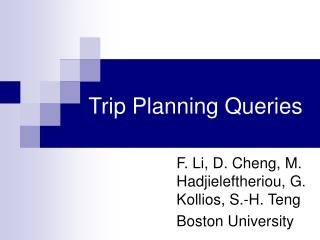DownloadDownload PresentationTrip Planning Queries

# Trip Planning Queries

Download Presentation## Trip Planning Queries

- - - - - - - - - - - - - - - - - - - - - - - - - - - E N D - - - - - - - - - - - - - - - - - - - - - - - - - - -
##### Presentation Transcript

1. Trip Planning Queries F. Li, D. Cheng, M. Hadjieleftheriou, G. Kollios, S.-H. Teng Boston University

2. Motivation • MapQuest, Google Maps, etc. have become essential web services. • Albeit, they provide simple driving directions given a start and an end point. • The same is true for vehicle navigation systems, GPS devices, etc… • It is time to support more advanced services!

3. A Novel Idea • Trip Planning Queries (TPQ): • Given a starting location, a destination and arbitrary points of interest try to find the best possible trip. • Example: • Minimize the total traveling time from Boston to Providence, while visiting a post office, a hardware store and a gas station.

4. Visual Example • We can minimize the total distance, time, etc. • We can have different categories of points of interest (gas stations, hotels, etc.). Home Work Gas station

5. Formally • Solve TPQ on metric graphs (e.g., transportation networks). • Given a metric graph G(V, E), a set Rof categories, a starting vertex S and an ending vertex D, find a vertex traversal T (or trip) from S to D that visits at least one vertex from each category in R and has the minimum possible cost. • Define the cost of a trip C(T) appropriately.

6. Observations • TPQ is harder than Traveling Salesman Problem (TSP): • Given any TSP instance assume that every vertex belongs to its own unique category. • To answer TPQ in practice we need to develop approximate solutions

7. The Nearest Neighbor Algorithm B2 A2 S D C2 B3 B1 C1 A1 • Yields a 2m+1 - 1 approximation where m is the total number of categories.

8. The Minimum Distance Algorithm B2 A2 S D C2 B3 B1 C1 A1 • Yields an m-approximation where m is the total number of categories.

9. p p 3 1 p 2 search region SR S D candidate p The Minimum Distance Algorithm • The Minimum Distance Algorithm restricts the search space/region as an ellipse.

10. Other Algorithms • Previous algorithms give approximations in terms of m. • Approximations in terms of the maximum category cardinality r: • Use Integer Linear Programming. • Approximations in terms of both m and r: • Use the generalized minimum spanning tree.

11. The Algorithms in Practice • Can we use the previous algorithms in practice for spatial databases? • Applications in Euclidean spaces using R-trees. • Applications in Road networks using specialized indices

12. NN Algorithm on R-trees • Starting from S locate the nearest neighbor of S that belongs to any category in R. • Iteratively continue until all categories have been covered. • Connect the last vertex found with D. • Use any NN algorithm on R-trees

13. MD Algorithm on R-trees • We need to locate one point per category such that L=|Sp| + |pD| is minimized. • We implement a NN search that tries to minimize L instead of MinDist when sorting the R-tree MBRs: B M D p A S

14. MD Algorithm on Road Network • As before, locate the m points that minimize the total network distance from S to D. • We implement a specialized algorithm for finding such points on a road network: • Expand all paths from S to D. • Separate into two categories: Paths that have located a point of interest p and ones that have not. • The first compete to find a shortest path to p. The latter compete to find a shortest path from p to D. • Return the path that minimizes the sum.

15. An Example in Road Network • We represent the point on a road network by its distance to the node with smaller index. n n 4 4 p (3.2) p (3.2) 2 2 p (3.2) 5.0 4 4.0 4.0 D(3.0) D(3.0) 5.0 4.0 4.0 p (0.8) p (0.8) 3 3 p (1.0) S(2.0) n n p (1.0) S(2.0) n n 1 2 1 1 2 6.0 1 6.0 n n 3 3 S->n2->p3->n2->D, 6.6 S->n2-> p4 ->D, 5.4

16. Experimental Evaluation • We used synthetic datasets on real road networks (Oldenburg) and real datasets from the state of California. • We varied the total number of categories m, the density per category r, and the network sizes. • We compare the NN and MD algorithms on road networks using R-trees.

17. Datasets

18. Average Trip Length

19. Query Cost

20. Conclusion • Introduced a novel query for spatial databases. • Designed four approximation algorithms with various approximation guarantees. • Implemented the algorithms in practice using R-trees for Euclidean spaces and road networks. • Contacted a comprehensive experimental evaluation.

21. Thank you!